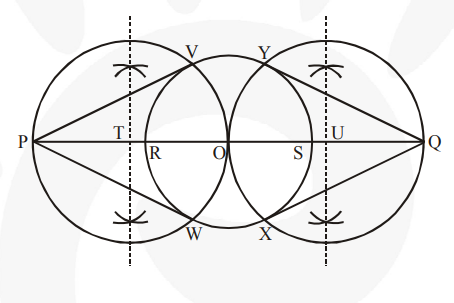# Draw a circle of radius 3 cm.`
Question:

Draw a circle of radius 3 cm. Take two points P and Q on one of its extended diameter each at a distance of 7 cm from its centre. Draw tangents to the circle from these two points P and Q.

Solution:

Steps of construction :

1. Taking any point O on the given plane as centre, draw a circle of 3 cm radius.

2. Take one of its diameters, RS, and extend it on both sides.

Locate two points on this diameter such that OP = OQ = 7 cm

3. Bisect OP and OQ. Let T and U be the mid-points of OP and OQ respectively.

4. Taking T and U as its centre and with TO and UO as radius, draw two circles. These two circles will intersect the circle at point V, W, X, Y respectively. Join PV, PW, QX, and QY. These are the required tangents.